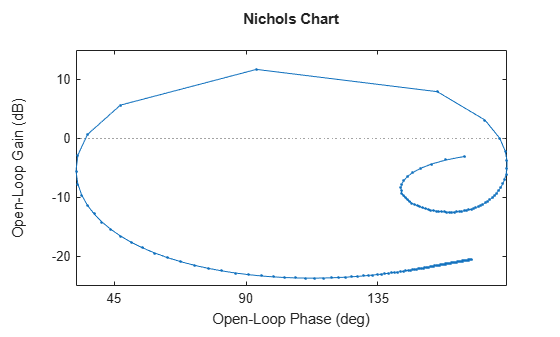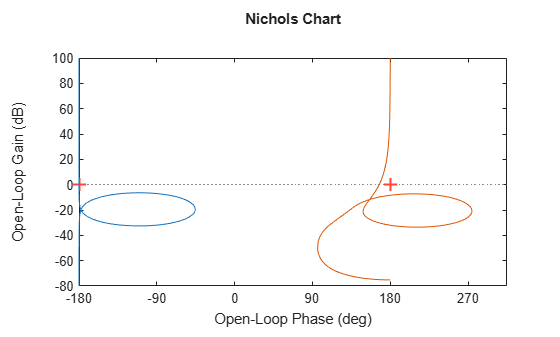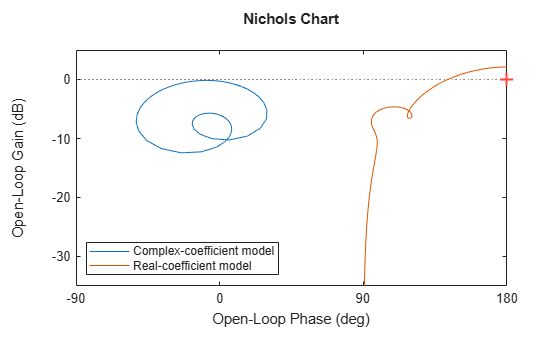# nichols

Nichols chart of frequency response

## Syntax

``nichols(sys)``
``nichols(sys1,sys2,...,sysN)``
``nichols(sys1,LineSpec1,...,sysN,LineSpecN)``
``nichols(___,w)``
``````[mag,phase,wout] = nichols(sys)``````
``````[mag,phase,wout] = nichols(sys,w)``````

## Description

example

````nichols(sys)` creates a Nichols chart of the frequency response of a dynamic system model `sys`. The plot displays the magnitude (in dB) and phase (in degrees) of the system response as a function of frequency. `nichols` automatically determines frequencies to plot based on system dynamics. Use `ngrid` to superimpose Nichols chart grid lines on an existing SISO Nichols chart.If `sys` is a multi-input, multi-output (MIMO) model, then `nichols` produces an array of Nichols charts, each plot showing the frequency response of one I/O pair.If `sys` is a model with complex coefficients, then `nichols` plot shows a contour comprised of both positive and negative frequencies. For models with real coefficients, `nichols` only shows positive frequencies.```

example

````nichols(sys1,sys2,...,sysN)` plots the Nichols plot of the frequency response of multiple dynamic systems on the same plot. All systems must have the same number of inputs and outputs.```

example

````nichols(sys1,LineSpec1,...,sysN,LineSpecN)` specifies a color, line style, and marker for each system in the plot.```

example

````nichols(___,w)` plots the response for frequencies specified by `w`.If `w` is a cell array of the form `{wmin,wmax}`, then `nichols` plots the chart at frequencies ranging between `wmin` and `wmax`.If `w` is a vector of frequencies, then `nichols` plots the chart at each specified frequency. The vector `w` can contain both negative and positive frequencies.You can use `w` with any of the input-argument combinations in previous syntaxes.```

example

``````[mag,phase,wout] = nichols(sys)``` returns the magnitude and phase of the response at each frequency in the vector `wout`. The function automatically determines frequencies in `wout` based on system dynamics. This syntax does not draw a plot.```

example

``````[mag,phase,wout] = nichols(sys,w)``` returns the response data at the frequencies specified by `w`.If `w` is a cell array of the form `{wmin,wmax}`, then `wout` contains frequencies ranging between `wmin` and `wmax`.If `w` is a vector of frequencies, then `wout` = `w`. ```

## Examples

collapse all

Plot the Nichols response with Nichols grid lines for the following system:

`$H\left(s\right)=\frac{-4{s}^{4}+48{s}^{3}-18{s}^{2}+250s+600}{{s}^{4}+30{s}^{3}+282{s}^{2}+525s+60}.$`

```H = tf([-4 48 -18 250 600],[1 30 282 525 60]); nichols(H) ngrid```Create a Nichols plot over a specified frequency range. Use this approach when you want to focus on the dynamics in a particular range of frequencies.

```H = tf([-0.1,-2.4,-181,-1950],[1,3.3,990,2600]); nichols(H,{1,100})```The cell array `{1,100}` specifies the minimum and maximum frequency values in the Nichols plot. When you provide frequency bounds in this way, the function selects intermediate points for frequency response data.

Alternatively, specify a vector of frequency points to use for evaluating and plotting the frequency response.

```w = 1:0.5:100; nichols(H,w,'.-')````nichols` plots the frequency response at the specified frequencies only.

Compare the frequency response of a continuous-time system to an equivalent discretized system on the same Nichols plot.

Create continuous-time and discrete-time dynamic systems.

```H = tf([1 0.1 7.5],[1 0.12 9 0 0]); Hd = c2d(H,0.5,'zoh');```

Create a Nichols plot that displays both systems.

`nichols(H,Hd)`Specify the line style, color, or marker for each system in a Nichols plot using the `LineSpec` input argument.

```H = tf([1 0.1 7.5],[1 0.12 9 0 0]); Hd = c2d(H,0.5,'zoh'); nichols(H,'r',Hd,'b--')```The first `LineSpec`, `'r'`, specifies a solid red line for the response of `H`. The second `LineSpec`, `'b--'`, specifies a dashed blue line for the response of `Hd`.

Compute the magnitude and phase of the frequency response of a SISO system.

If you do not specify frequencies, `nichols` chooses frequencies based on the system dynamics and returns them in the third output argument.

```H = tf([1 0.1 7.5],[1 0.12 9 0 0]); [mag,phase,wout] = nichols(H);```

Because `H` is a SISO model, the first two dimensions of `mag` and `phase` are both 1. The third dimension is the number of frequencies in `wout`.

`size(mag)`
```ans = 1×3 1 1 110 ```
`length(wout)`
```ans = 110 ```

Thus, each entry along the third dimension of `mag` gives the magnitude of the response at the corresponding frequency in `wout`.

For this example, create a 2-output, 3-input system.

```rng(0,'twister'); H = rss(4,2,3);```

For this system, `nichols` plots the frequency responses of each I/O channel in a separate plot in a single figure.

`nichols(H)`Compute the magnitude and phase of these responses at 20 frequencies between 1 and 10 radians.

```w = logspace(0,1,20); [mag,phase] = nichols(H,w);```

`mag` and `phase` are three-dimensional arrays, in which the first two dimensions correspond to the output and input dimensions of `H`, and the third dimension is the number of frequencies. For instance, examine the dimensions of `mag`.

`size(mag)`
```ans = 1×3 2 3 20 ```

Thus, for example, `mag(1,3,10)` is the magnitude of the response from the third input to the first output, computed at the 10th frequency in `w`. Similarly, `phase(1,3,10)` contains the phase of the same response.

Create a Nichols plot of a model with complex coefficients and a model with real coefficients on the same plot.

```rng(0) A = [-3.50,-1.25-0.25i;2,0]; B = [1;0]; C = [-0.75-0.5i,0.625-0.125i]; D = 0.5; Gc = ss(A,B,C,D); Gr = rss(7); nichols(Gc,Gr) legend('Complex-coefficient model','Real-coefficient model','Location','southwest')```For models with complex coefficients, `nichols` shows a contour comprised of both positive and negative frequencies. For models with real coefficients, the plot shows only positive frequencies, even when complex-coefficient models are present. You can click the curve to further examine which section and values correspond to positive and negative frequencies.

## Input Arguments

collapse all

Dynamic system, specified as a SISO or MIMO dynamic system model or array of dynamic system models. Dynamic systems that you can use include:

• Continuous-time or discrete-time numeric LTI models, such as `tf`, `zpk`, or `ss` models.

• Generalized or uncertain LTI models such as `genss` or `uss` (Robust Control Toolbox) models. (Using uncertain models requires Robust Control Toolbox™ software.)

• For tunable control design blocks, the function evaluates the model at its current value for both plotting and returning frequency response data.

• For uncertain control design blocks, the function plots the nominal value and random samples of the model. When you use output arguments, the function returns frequency response data for the nominal model only.

• Frequency-response data models such as `frd` models. For such models, the function plots the response at frequencies defined in the model.

• Identified LTI models, such as `idtf` (System Identification Toolbox), `idss` (System Identification Toolbox), or `idproc` (System Identification Toolbox) models. Using identified models requires System Identification Toolbox™ software.

If `sys` is an array of models, the function plots the frequency responses of all models in the array on the same axes.

Line style, marker, and color, specified as a string or vector of one, two, or three characters. The characters can appear in any order. You do not need to specify all three characteristics (line style, marker, and color). For example, if you omit the line style and specify the marker, then the plot shows only the marker and no line. For more information about configuring this argument, see the `LineSpec` input argument of the `plot` function.

Example: `'r--'` specifies a red dashed line

Example: `'*b'` specifies blue asterisk markers

Example: `'y'` specifies a yellow line

Frequencies at which to compute and plot frequency response, specified as the cell array `{wmin,wmax}` or as a vector of frequency values.

• If `w` is a cell array of the form `{wmin,wmax}`, then the function computes the response at frequencies ranging between `wmin` and `wmax`.

• If `w` is a vector of frequencies, then the function computes the response at each specified frequency. For example, use `logspace` to generate a row vector with logarithmically spaced frequency values. The vector `w` can contain both positive and negative frequencies.

For models with complex coefficients, if you specify a frequency range of [wmin,wmax] for your plot, then the plot shows a contour comprised of both positive frequencies [wmin,wmax] and negative frequencies [–wmax,–wmin].

Specify frequencies in units of rad/`TimeUnit`, where `TimeUnit` is the `TimeUnit` property of the model.

## Output Arguments

collapse all

Magnitude of the system response in absolute units, returned as a 3-D array. The dimensions of this array are (number of system outputs) × (number of system inputs) × (number of frequency points).

To convert the magnitude from absolute units to decibels, use:

```magdb = 20*log10(mag) ```

Phase of the system response in degrees, returned as a 3-D array. The dimensions of this array are (number of outputs)-by-(number of inputs)-by-(number of frequency points).

Frequencies at which the function returns the system response, returned as a column vector. The function chooses the frequency values based on the model dynamics, unless you specify frequencies using the input argument `w`.

`wout` also contains negative frequency values for models with complex coefficients.

Frequency values are in radians/`TimeUnit`, where `TimeUnit` is the value of the `TimeUnit` property of `sys`.

## Version History

Introduced before R2006a